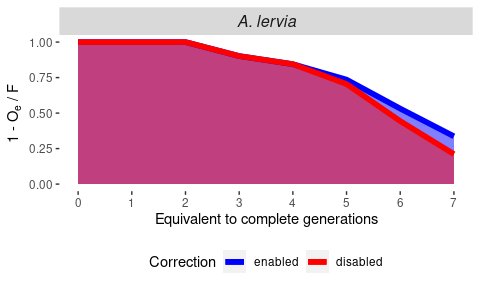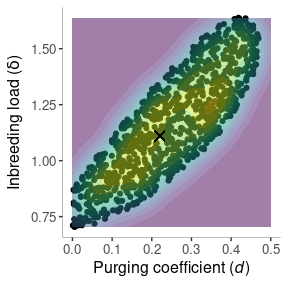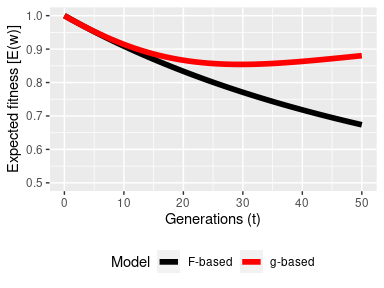Inbreeding and Purging Estimates

2021-12-13

This vignette provides a practical guide on how to use functions in purgeR to estimate parameters related to inbreeding and purging using estimates obtained with functions from purgeR. For illustrative purposes, we will estimate here the magnitude of the inbreeding load ($$B$$) and the purging coefficient ($$d$$) using different approaches. Note however that this guide is not exhaustive. Different methods to those applied here could be used to estimate these parameters, as well as alternative models of inbreeding and purging. For example, purging models could be based on ancestral inbreeding (e.g. Boakes et al. 2007) or the individual reduction in inbreeding load (e.g. Gulisija and Crow 2007). If you haven’t, read the ‘purgeR-tutorial’ vignette for a more concise and complete introduction to functions in purgeR.

The inbreeding load plays a determinant role in the survivability of small populations, and estimating its potential reduction is one of the main aims of genetic purging models. Following Gulisija and Crow (2007), the expected individual reduction in the inbreeding load component ascribed to high effect size, deleterious mutations, can be estimated from the expressed opportunity of purging ($$O_{e}$$), and normalized by the level of inbreeding ($$O_{e}/F$$, Gulisija and Crow 2007).

Using the function ip_op(), only the pedigree structure is required to estimate these parameters. Taking the pedigree of the Barbary sheep (A. lervia) as an example, we compute the proportional reduction in inbreeding load ($$B_{t=0}$$) with generations as $$(1-O_{e}/F$$), so that at time $$t$$, the expected $$B = B_{t=0}(1-O_{e}/F)$$.

data(arrui)
arrui <- arrui %>%
purgeR::ip_F() %>%
purgeR::ip_op(Fcol = "Fi") %>%
dplyr::mutate(species = "A. lervia") %>%
purgeR::pop_t() %>%
dplyr::mutate(t = plyr::round_any(t, 1))
#> Computing partial kinship matrix. This may take a while.

arrui %>%
dplyr::group_by(species, t) %>%
dplyr::summarise(Fi = mean(Fi),
Oe = mean(Oe),
Oe_raw = mean(Oe_raw),
nOe = ifelse(Fi > 0, Oe/Fi, 0),
nOe_raw = ifelse(Fi > 0, Oe_raw/Fi, 0)) %>%
ggplot(aes(x = t)) +
geom_area(aes(y = 1-nOe), fill = "blue", alpha = 0.5) +
geom_area(aes(y = 1-nOe_raw), fill = "red", alpha = 0.5) +
geom_line(aes(y = 1-nOe, color = "enabled"), size = 2) +
geom_line(aes(y = 1-nOe_raw, color = "disabled"), size = 2) +
facet_grid(. ~ species) +
scale_y_continuous(expression(paste("1 - ", O["e"], " / F", sep = "")), limits = c(0, 1)) +
scale_x_continuous("Equivalent to complete generations", breaks = c(0,1,2,3,4,5,6,7)) +
scale_color_manual("Correction", values = c(enabled  = "blue", disabled = "red")) +
theme(panel.background = element_blank(),
strip.text = element_text(size = 12, face = "italic"),
legend.position = "bottom")
#> summarise() has grouped output by 'species'. You can override using the .groups argument.The plots shows two different estimations of $$O_e$$. In blue, the default estimate enables the correction required for $$O_{e}$$ for complex pedigrees (Gulisija and Crow 2007), using the heuristic proposed by López-Cortegano (2021). In red, $$O_{e}$$ is estimated without correction terms (returned as ‘Oe_raw’ by ip_op()). It is expected that the actual reduction in inbreeding load ranges between the two estimates (see López-Cortegano 2021 for more exhaustive examples using populations simulated under different mutational models). Thus, in this case the figure suggest that in the last cohort analyzed in A. lervia, the inbreeding load of highly deleterious recessive mutations could have been reduced between 66 % and 79 % from its original value.

Note that models based on purged inbreeding also allow to estimate inbreeding load decline (García-Dorado 2012), but these will require precise estimates of fitness and of the purging coefficient. Estimation of purging coefficients under this model is shown in sections below.

Fitness change under inbreeding and purging

In sections below, we assume Morton et al. (1956) multiplicative model of fitness to fit and predict inbreeding and purging models, and some examples are used in sections below. Under this model, the expected fitness ($$E(W)$$) can be calculated as:

$E(W) = W_{0} \cdot e^{BF+AX}$ Where $$B$$ is the inbreeding depression rate, $$F$$ is the inbreeding coefficient, and $$A$$ and $$X$$ represent additional regression coefficient and predictor terms associated with other possible factors affecting fitness (e.g. environmental effects).

Estimating inbreeding depression

Using logarithms, the expression above becomes the equation of a line, which can be solved by simple linear regression:

$log[E(W)] = log(W_{0}) + BF + AX$ Taking Dama gazelle (N. dama) as an example pedigree, we could use this model to estimate the inbreeding depression rate on female productivity as follows:

data(dama)
dama %>%
purgeR::ip_F() %>%
dplyr::filter(prod > 0) %>%
stats::lm(formula = log(prod) ~ Fi)
#>
#> Call:
#> stats::lm(formula = log(prod) ~ Fi, data = .)
#>
#> Coefficients:
#> (Intercept)           Fi
#>       1.763       -1.471

Note however the limitation that values of fitness equal to zero have to be removed. This can be a problem for binary fitness traits such as survival. Fortunately, R provides a fairly complete set of statistic methods, and a logistic regression approach could be used in this case:

dama %>%
purgeR::ip_F() %>%
stats::glm(formula = survival15 ~ Fi, family = "binomial")
#>
#> Call:  stats::glm(formula = survival15 ~ Fi, family = "binomial", data = .)
#>
#> Coefficients:
#> (Intercept)           Fi
#>       1.826       -3.139
#>
#> Degrees of Freedom: 1010 Total (i.e. Null);  1009 Residual
#>   (305 observations deleted due to missingness)
#> Null Deviance:       1148
#> Residual Deviance: 1141  AIC: 1145

Working with more complex models

The same principle can be applied to more complex models that include terms associated with inbreeding (like $$F$$ in previous examples), but also others associated with genetic purging, and environmental factors. Similarly, different statistical methods can be applied, making the most of R extensive package library.

One remarkable example is making use of the Classification And REgression Training (caret) R package. Providing more complex and exhaustive examples using this library falls out of the scope of this tutorial, but as a naive example, a regularized logistic regression method is used below to fit a training set with N. dama data, and estimate regression coefficient terms on a model including standard and ancestral inbreeding as genetic factors, plus year of birth and period of management as environmental factors. The confusion matrix shows that the model has little predictive value though.

set.seed(1234)
vars <- c("survival15", "Fi", "Fa", "yob", "pom")
df <- dama %>%
purgeR::ip_F() %>%
purgeR::ip_Fa(Fcol = "Fi") %>%
dplyr::select(tidyselect::all_of(vars)) %>%
stats::na.exclude()
trainIndex <- caret::createDataPartition(df$survival15, p = 0.75, list = FALSE, times = 1) df_train <- df[trainIndex, ] df_test <- df[-trainIndex, ] m <- caret::train(factor(survival15) ~., data = df_test, method = "glmnet") stats::predict(m$finalModel, type = "coefficients", s = m$bestTune$lambda)
#> 5 x 1 sparse Matrix of class "dgCMatrix"
#>                     s1
#> (Intercept)  2.2594269
#> Fi          -4.8140731
#> Fa          -0.1841322
#> yob          .
#> pomvet       .
stats::predict(m, df_test) %>%
caret::confusionMatrix(reference = factor(df_test$survival15)) #> Confusion Matrix and Statistics #> #> Reference #> Prediction 0 1 #> 0 2 0 #> 1 65 185 #> #> Accuracy : 0.7421 #> 95% CI : (0.6834, 0.7949) #> No Information Rate : 0.7341 #> P-Value [Acc > NIR] : 0.4195 #> #> Kappa : 0.0432 #> #> Mcnemar's Test P-Value : 2.051e-15 #> #> Sensitivity : 0.029851 #> Specificity : 1.000000 #> Pos Pred Value : 1.000000 #> Neg Pred Value : 0.740000 #> Prevalence : 0.265873 #> Detection Rate : 0.007937 #> Detection Prevalence : 0.007937 #> Balanced Accuracy : 0.514925 #> #> 'Positive' Class : 0 #> Estimating the purging coefficient: Regression examples Models based on purged inbreeding ($$g$$), require a value of the purging coefficient ($$d$$) to be estimated or assumed. In this case, it is possible to iterate over a range of candidate values of $$d$$, and fit the different regression model alternatives, to finally retain the one providing the best fit. Code below shows how to do this assuming logistic regression for early survival in N. dama data: d_values <- seq(from = 0.0, to = 0.5, by = 0.01) models <- seq_along(d_values) %>% purrr::map(~ip_g(ped = dama, d = d_values[[.]], name_to = "g")) %>% purrr::map(~glm(formula = survival15 ~ g, family = binomial(link = "probit"), data = .)) aic_values <- models %>% purrr::map(summary) %>% purrr::map_dbl("aic") aic_best <- aic_values %>% min() models[[which(aic_values == aic_best)]] %>% summary #> #> Call: #> glm(formula = survival15 ~ g, family = binomial(link = "probit"), #> data = .) #> #> Deviance Residuals: #> Min 1Q Median 3Q Max #> -2.0147 -1.3141 0.7404 0.7616 1.1577 #> #> Coefficients: #> Estimate Std. Error z value Pr(>|z|) #> (Intercept) 1.1198 0.1535 7.294 3e-13 *** #> g -2.5950 0.8243 -3.148 0.00164 ** #> --- #> Signif. codes: 0 '***' 0.001 '**' 0.01 '*' 0.05 '.' 0.1 ' ' 1 #> #> (Dispersion parameter for binomial family taken to be 1) #> #> Null deviance: 1148.4 on 1010 degrees of freedom #> Residual deviance: 1138.3 on 1009 degrees of freedom #> (305 observations deleted due to missingness) #> AIC: 1142.3 #> #> Number of Fisher Scoring iterations: 4 The estimated purging coefficient $$d = 0.19$$ here is close to that obtained by López-Cortegano et al. (2021) using a heuristic approach, but it is a convenient example because it reminds of some of the limitations of using transformations on Morton’s model, e.g. due to Jensen’s inequality when linearized with logarithms (see details in García-Dorado et al. 2016). Thus, it is better practice to fit this model in the original scale of the data, using exponential, non-linear regression methods. This use is illustrated below using R nls function: set.seed(1234) d_values <- seq(from = 0.0, to = 0.5, by = 0.01) start_values <- seq_along(d_values) %>% map(~ip_g(ped = dama, d = d_values[[.]], name_to = "g")) %>% map(~glm(formula = survival15 ~ g, family = binomial(link = "probit"), data = .)) %>% map("coefficients") %>% map(~set_names(x = ., nm = c("W0", "B"))) models <- seq_along(d_values) %>% map(~ip_g(ped = dama, d = d_values[[.]], name_to = "g")) %>% map2(start_values, ~nls(formula = survival15 ~ W0 * exp(B * g), start = .y, data = .x)) aic_values <- models %>% map_dbl(AIC) aic_best <- aic_values %>% min() models[[which(aic_values == aic_best)]] %>% summary #> #> Formula: survival15 ~ W0 * exp(B * g) #> #> Parameters: #> Estimate Std. Error t value Pr(>|t|) #> W0 0.89658 0.05821 15.402 < 2e-16 *** #> B -1.11225 0.38305 -2.904 0.00377 ** #> --- #> Signif. codes: 0 '***' 0.001 '**' 0.01 '*' 0.05 '.' 0.1 ' ' 1 #> #> Residual standard error: 0.4343 on 1009 degrees of freedom #> #> Number of iterations to convergence: 5 #> Achieved convergence tolerance: 2.829e-06 #> (305 observations deleted due to missingness) d_values[which(aic_values == aic_best)] #>  0.22 This new estimate avoids problems derived from scale transformation. For example, the intercept (0.897) representing the expected fitness of non-inbred individuals is closer to the actual fitness of non-inbred individuals in the pedigree (0.745). Similarly, the estimate of the inbreeding depression rate ($$B = -1.112$$) and the purging coefficient ($$d = 0.22$$) are closer to previously estimated values in this population using PURGd (García-Dorado et al. 2016). Note however, that purgeR accuracy and performance when estimating $$d$$ is superior to that of PURGd (López-Cortegano 2021). Estimating the purging coefficient: Bayesian example Above we have used regression methods to obtain maximum likelihood estimates of different inbreeding and purging parameters, but we could also take a Bayesian approach using R functions, and ask about the posterior distribution of some of these parameters. Below, we focus on the re-estimation of the inbreeding load and the purging coefficient using Approximate Bayesian Computation (ABC). Again, this is intended to provide an example of use, and we warn that the summary statistics used as well as the threshold value have not been extensively tested. A good review on ABC methods and best practices can be found in Beaumont (2010). # Initialize observed data data(dama) dama <- dama %>% ip_F() w0 <- 0.89658 # assumed fitness for non-inbred individuals, from regression above # We use fitness itself as summary statistic (So) # In the simulated data, fitness (Ss) is computed using Morton et al. model # Distance between So and Ss [d(So, Ss)] is estimated by means of the residual sum of squares (RSS) get_RSS <- function(data, par) { data %>% purgeR::ip_g(d = par, Fcol = "Fi", name_to = "g") %>% dplyr::mutate(Ew = w0 * exp(-par * g)) %>% dplyr::filter(!is.na(survival15)) %$%
-(survival15-Ew) %>%
^(2) %>%
sum()
}

# Acceptance rule for d(so, Ss) given a threshold
ABC_accept <- function(data, par, threshold){
if (par < 0) return(FALSE)
if (par > 0.5) return(FALSE)
if (par < 0) return(FALSE)
if(RSS < threshold) return(TRUE) else return(FALSE)
}

# Run MCMC ABC
MCMC_ABC <- function(data, niter, nburn, nthin, threshold) {
nsamples <- (niter-nburn)/nthin
chain <- array(dim = c(nsamples, 3)) # d, delta and RSS
sample <- c(0.0, 0.0, get_RSS(data, c(0.0, 0.0)))
sample_idx <- 1
for (i in 1:niter) {
d_test <- runif(1, min = 0, max = 0.5)
delta_test <- runif(1, min = 0, max = 15)
sample_test <- c(d_test, delta_test, get_RSS(data, c(d_test, delta_test)))

if (ABC_accept(data, sample_test, threshold)) sample <- sample_test
if ((i > nburn) & (i %% nthin == 0)) {
print(sample_idx)
chain[sample_idx,] <- sample
sample_idx <- sample_idx + 1
}
}
return(coda::mcmc(chain, start = nburn, thin = nthin))
}

# Get the posterior
set.seed(1234)
# We set an arbitrary threshold taking the RSS from the best fit
# and allowing an increase in the simulations up to an 0.5% in value
t = t*1.005
# Get the posterior distribution for the purging coefficient and delta
# A third column with the RSS values is also returned
posterior <- MCMC_ABC(dama, niter = 5100, nburn = 100, nthin = 50, threshold = t*1.005)

We could now make some basic checks on the MCMC chain generated, including convergence and auto-correlation tests. In this case, the generated MCMC chain passes Heidelberger and Welch’s convergence test, that auto-correlation is low given the thinning interval used, and that the effective number of samples is close to 1000 (code not run here).

posterior[, 1:2] %>% base::plot()
posterior[, 1:2] %>% coda::heidel.diag()
posterior[, 1:2] %>% coda::autocorr.diag()
posterior[, 1:2] %>% coda::effectiveSize()

Finally, the joint posterior distribution of the two estimated parameters, as well as their marginal distributions:

df <- data.frame(posterior)

# Joint posterior distribution
ggplot(data = df) +
geom_point(aes(x = d, y = delta)) +
geom_density_2d_filled(aes(x = d, y = delta), alpha = 0.5) +
geom_point(x = 0.22, y = 1.11, size = 3, shape = 4) +
scale_x_continuous(expression(paste("Purging coefficient (", italic(d), ")", sep = "")),
limits = c(0, 0.5)) +
scale_y_continuous(expression(paste("Inbreeding load (", italic(delta), ")", sep = "")),
limits = c(min(df$delta), max(df$delta))) +
theme(panel.background = element_blank(),
axis.line = element_line(size = 0.1),
axis.title = element_text(size = 12),
axis.text = element_text(size = 10),
legend.position = "none")The plot above shows that the maximum likelihood estimate of $$B$$ and $$d$$ obtained above (marked with an X) falls within the joint distribution of the two parameters as expected. However, while the distribution of $$d$$ covers almost all the possible range of values [0.0, 0.5], we have better evidence that $$B$$ takes values below $$B<2$$ which can be considered a low value in agreement with previous estimates of this parameter in wild populations (O’Grady et al. 2006). In addition, we observe a linear association between $$B$$ and $$d$$, suggesting that if the actual purging coefficient estimated in the population were below the assumed maximum likelihood estimate of 0.22, the inbreeding load estimate would also be lower, indicating in all cases that generally the inbreeding load is expected to be largely purged in this population, and that fitness depression will be much lower than expected disregarding purging.

Fitness prediction

We have used Morton et al (1956) model to compute predictions on the expected fitness conditional to estimates of the inbreeding load and purged inbreeding (and the purging coefficient). Below a plot is shown assuming $$B=1$$, $$N_{e} = 50$$ and fitness in the base population $$W_{t=0}=1$$. The black line represent the classical prediction of inbreeding depression based on $$F$$ increase, and the red one is a prediction using $$g(d=0.10)$$ for illustrative purposes.

w0 <- 1
B <- 1
data.frame(t = 0:50) %>%
dplyr::rowwise() %>%
dplyr::mutate(Fi = exp_F(Ne = 50, t),
g = exp_g(Ne = 50, t, d = 0.10),
exp_WF = w0*exp(-B*Fi),
exp_Wg = w0*exp(-B*g)) %>%
tidyr::pivot_longer(cols = c(exp_WF, exp_Wg), names_to = "Model", values_to = "Ew") %>%
ggplot(aes(x = t, y = Ew, color = Model)) +
geom_line(size = 2) +
scale_x_continuous("Generations (t)") +
scale_y_continuous("Expected fitness [E(w)]", limits = c(0.5, 1)) +
scale_color_manual(labels = c("F-based", "g-based"), values = c("black", "red")) +
theme(legend.position = "bottom")It is remarkable that even small $$d$$ estimates result in expectations of important fitness recovery. Hence, the importance of considering genetic purging theory in problems related to evolutionary biology and conservation.